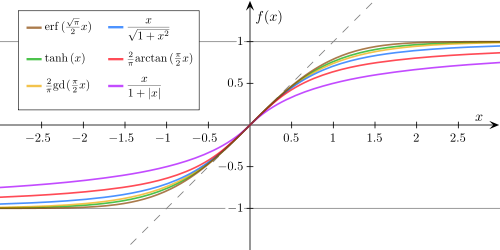-->

## Thursday, January 31, 2013

### A Sigmoid Function Without the Exponential: Elliot Sigmoid Activation FunctionToday I learned about the Elliot Activation (or Sigmoid) Function. In fact, The MathWorks just included it in their most recent update to the Neural Network toolbox. The function was first introduced in 1993 by D.L. Elliot under the title A Better Activation Function for Artificial Neural Networks. The function closely approximates the Sigmoid or Hyperbolic Tangent functions for small values, however it takes longer to converge for large values (i.e. It doesn't go to 1 or 0 as fast), though this isn't particularly a problem if you're using it for classification.

In my testing in MATLAB "the function computes over 2x faster than the exponential sigmoid function", which, for certain types of ML problems can lead to a significant speed improvement. So what is this function?

Behold:

\begin{aligned} \sigma_e(x) = \frac{1}{1 + |x|} \end{aligned}

It's also differntiable with the derivative of

\begin{aligned} \frac{\partial\sigma_e(x)}{\partial x} = \frac{1}{(1 + |x|)^2} \end{aligned}

For an activation function in the range $[0,1]$ it can be written as

\begin{aligned} \sigma_e(x) = \frac{0.5(x)}{1 + |x|} + 0.5 \end{aligned}

In MATLAB this can be simply written as

function p = elliotsig(x)
% From http://citeseerx.ist.psu.edu/viewdoc/summary?doi=10.1.1.46.7204
% "A better Activation Function for Artificial Neural Networks"

p = 0.5*(x)/(1 + abs(x))+0.5;

end


Here's an image I ganked from Wikipedia that shows the Elliot, Exponential and Hyperbolic Tangent Sigmoid functions: